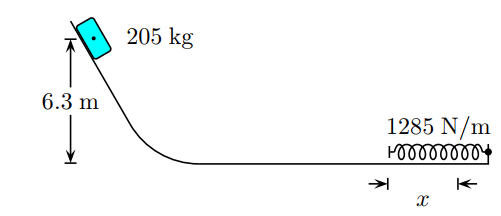Problem: A 205 kg block is released at a 6.3 m height as shown. The track is frictionless. The block travels down the track, hits a spring of force constant k = 1285 N/m . The acceleration of gravity is 9.8 m/s2. Determine the compression of the spring x from its equilibrium position before coming to rest momentarily. A. 3.39911 B. 2.46691 C. 3.05595 D. 2.80797 E. 3.4477 F. 3.57838 G. 2.50776 H. 3.66395 I. 3.72911 J. 4.43837

FREE Expert Solution
99% (290 ratings)
Problem Details

A 205 kg block is released at a 6.3 m height as shown. The track is frictionless. The block travels down the track, hits a spring of force constant k = 1285 N/m . The acceleration of gravity is 9.8 m/s2.

Determine the compression of the spring x from its equilibrium position before coming to rest momentarily.

A. 3.39911

B. 2.46691

C. 3.05595

D. 2.80797

E. 3.4477

F. 3.57838

G. 2.50776

H. 3.66395

I. 3.72911

J. 4.43837